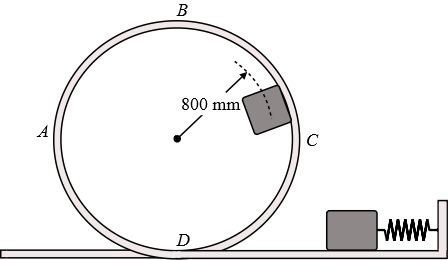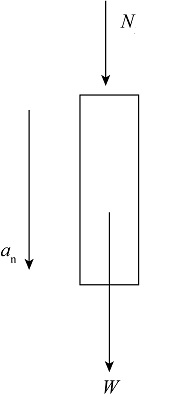# A 0.3 kg block is released from rest after a spring of constant k = 600 N/m has been compressed...

## Question:

A 0.3 kg block is released from rest after a spring of constant k = 600 N/m has been compressed 160 mm. The block is not attached to the spring but is simply pushed against it. The block travels in a vertical plane when released. Determine the speed of the block as it passes point B and the force exerted by the loop ABCD on the block as it passes through point B. Assume no friction.## Normal Acceleration:

Normal acceleration is the acceleration which comes in the picture due to change in the direction of velocity. Its direction always acts towards the center of the circle.

## Answer and Explanation:

Given Data

• The mass of the block is :{eq}m = 0.3\;{\rm{kg}} {/eq}
• The spring constant is: {eq}k = 600\;{\rm{N/m}} {/eq}
• The length up to which spring is compressed is :{eq}x = 160\;{\rm{mm}} = {\rm{0}}{\rm{.16}}\;{\rm{m}} {/eq}
• The distance of center of block from the center of block is :{eq}h = 800\;{\rm{mm}} = {\rm{0}}{\rm{.8}}\;{\rm{m}} {/eq}

Apply conservation of energy to find the velocity of block just after it released by the spring.

{eq}\dfrac{1}{2}mv_1^2 = \dfrac{1}{2}k{x^2} {/eq}

Substitute all the values in the above equation.

{eq}\begin{align*} \dfrac{1}{2}\left( {0.3\;{\rm{kg}}} \right)v_1^2 &= \dfrac{1}{2}\left( {600\;{\rm{N/m}}} \right){\left( {0.\;16{\rm{m}}} \right)^2}\\ \left( {0.3\;{\rm{kg}}} \right)v_1^2 &= 192\;{\rm{N}} \cdot {\rm{m}}\left( {\dfrac{{{\rm{kg}} \cdot {{\rm{m}}^{\rm{2}}}{\rm{/}}{{\rm{s}}^{\rm{2}}}}}{{{\rm{N}} \cdot {\rm{m}}}}} \right)\\ {v_1}& = 7.15\;{\rm{m/s}} \end{align*} {/eq}

Apply conservation to find the velocity of block at point B.

{eq}k.{E_1} = k.{E_2} + P.{E_2} {/eq}

Here, kinetic energy of block at point D is kinetic energy of block at point B is {eq}k.{E_2} {/eq} and potential energy of block at point D is {eq}P.{E_2} {/eq}.

Substitute all the expression in the above equation.

{eq}\dfrac{1}{2}mv_1^2 = \dfrac{1}{2}mv_2^2 + mg\left( {2h} \right) {/eq}

Here, velocity at B is {eq}{v_2} {/eq}.

Substitute all the values in the above equation.

{eq}\begin{align*} \dfrac{1}{2}\left( {0.3\;{\rm{kg}}} \right){\left( {7.15\;{\rm{m/s}}} \right)^2} &= \dfrac{1}{2}\left( {0.3\;{\rm{kg}}} \right)v_2^2 + \left( {0.3\;{\rm{kg}}} \right)\left( {9.81\;{\rm{m/}}{{\rm{s}}^{\rm{2}}}} \right)\left( {2\left( {0.8\;{\rm{m}}} \right)} \right)\\ {v_2}& = 4.44\;{\rm{m/s}} \end{align*} {/eq}

Thus the velocity of block at point B is {eq}4.44\;{\rm{m/s}} {/eq}.

The figure below shows the free body diagram of block at point B.The normal acceleration of block at B point is,

{eq}{a_n} = \dfrac{{v_2^2}}{h} {/eq}

Substitute all the values in the above equation.

{eq}\begin{align*} {a_n} &= \dfrac{{{{\left( {4.44\;{\rm{m/s}}} \right)}^2}}}{{0.8\;{\rm{m}}}}\\ {a_n} &= 24.64\;{\rm{m/}}{{\rm{s}}^{\rm{2}}} \end{align*} {/eq}

The weight of the block is,

{eq}W = mg {/eq}

Substitute all the values in the above equation.

{eq}\begin{align*} W &= 0.3\;{\rm{kg}} \times \left( {9.81\;{\rm{m/}}{{\rm{s}}^{\rm{2}}}} \right)\\ W&= 2.943\;{\rm{kg}} \cdot {\rm{m/}}{{\rm{s}}^{\rm{2}}}\left( {\frac{{\rm{N}}}{{{\rm{kg}} \cdot {\rm{m/}}{{\rm{s}}^{\rm{2}}}}}} \right)\\ W&= 2.943\;{\rm{N}} \end{align*} {/eq}

Apply Newton's second law.

{eq}N + W = m{a_n} {/eq}

Here, {eq}N {/eq} is the normal force applied by the loop on the block.

Substitute all the values in the above equation.

{eq}\begin{align*} N +2.943\;{\rm{N}} & = \left( {0.3\;{\rm{kg}}} \right)24.64\;{\rm{m/}}{{\rm{s}}^{\rm{2}}}\\ N &= 4.44\;{\rm{N}} \end{align*} {/eq}

Thus normal force applied by the loop on the block is {eq}4.44\;{\rm{N}} {/eq}.